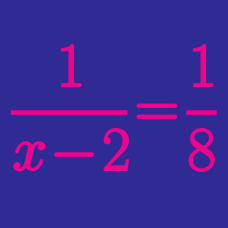Algebra

# Square roots

Evaluate

$\sqrt{ 25 } .$

Which of the following is the positive square root of 144?

NOTE: The notation $\sqrt{144}$ means the same thing; that is, it indicates by default the positive square root. If both roots are desired the notation $\pm \sqrt{144}$ is used.

What is the positive square root of 1?

What is the positive square root of 9?

The positive square root of a number $X$ is 4. What is $X$?

×